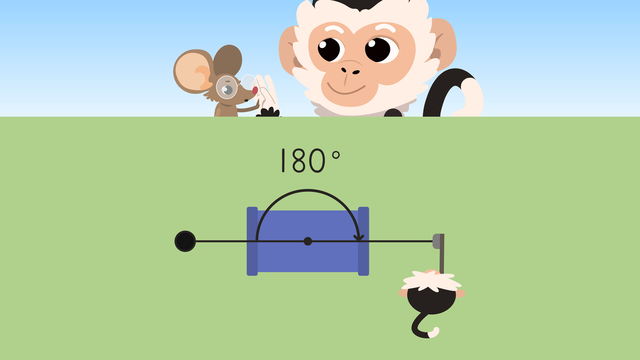# Drawing AnglesRate this video

Ø 5.0 / 1 ratings

The authorTeam Digital
Drawing Angles
CCSS.MATH.CONTENT.4.MD.C.6

## Information about the videoDrawing Angles

### Contents

#### In this Video

Nico and Nia are playing mini golf. Nico is figuring out the angle he needs to hit the ball in order to get a hole in one. We can help

### TranscriptDrawing Angles

Nico and Nia are playing mini-golf. Nico is figuring out the angle he needs to hit the ball in order to get a hole in one. If he understands the angles, he can get the perfect putt. : “Drawing Angles” A protractor is the tool we use to make angles. The baseline helps draw straight lines and line up the angles. The inner and outer scales are what we use to measure the degrees. REMEMBER, we use the set of numbers on the scale that are facing the SAME DIRECTION as our angle opening. Let’s see how we can help Nico use the protractor to draw the angle as he lines up his putt. If he putts a one hundred eighty-degree straight angle through the tunnel, he will make this shot. First, he'll use the tunnel as the MIDDLE of the angle and make a dot here for the VERTEX. Next, he'll use the straight edge to draw a LINE SEGMENT from the vertex to the ball and from the vertex to the cup. Nico swings and(...)HOLE IN ONE! WE DID IT! This next course is challenging because he must hit the ball off the statue to go into the cup. Using the statue as the point where the line segments meet, let’s help Nico draw this angle. What should we do first? First, we’ll make a dot to represent the vertex. Then we need to line up the vertex and the ball. Draw a line segment to connect these two points. Now line this up with the zero on the OUTER SCALE of the protractor. Follow the outer scale until it intersects with the cup. How many degrees is this angle? This angle is ONE HUNDRED FIFTEEN degrees. Make a dot at this point just above the protractor. Move the protractor so the straight edge lines up from the vertex to the mark(...) made at one hundred fifteen degrees. Draw a line segment to connect these two points. If Nico follows our angle, he will make the hole in one! YES! This next hole looks like a right angle. Let's draw it to see if it is ninety degrees. We'll use the pinwheel as the vertex. First, make a dot here. Using the straight edge, draw a line segment from the vertex to the ball. Now we will line up with the zero on the outer scale. What do we need to do next? We follow the outer scale until it intersects with the cup. Here, we make a dot above the protractor at NINETY degrees. Now we return to the straight edge and line it up from the vertex to this point. We'll draw a straight line to connect. Did we make a right angle? Nico's putt follows the right angle and he has DONE it again! The last hole of the game is a thirty-degree angle. Let’s draw it so Nico knows what to do. The tower of the castle is where the two line segments meet, so we’ll draw the dot there to show the vertex. Draw the line segment from the vertex to the ball. What should we do next? Place the baseline along this line segment where it lines up with zero. On the outer scale, look for thirty degrees Place a mark at the thirty-degree point because this is where the cup intersects. Then we’ll use the straight edge to connect these points. Remember(...)we can use a protractor to draw angles. First, make a vertex and use the straight edge to draw a line. Then, place the center of the baseline on the vertex and line up with zero. Next, find the given measurement along the proper scale and mark that spot. Finally, use the straight edge to connect the vertex to the measured spot. [Nico hits the ball into the hole and the ball pops back out. Nico looks confused and looks into the hole; Nia is in there. She hops up and high fives Nico.] Nico scored a perfect game by drawing angles to figure out how he should hit the ball! Now THAT deserves a high five!